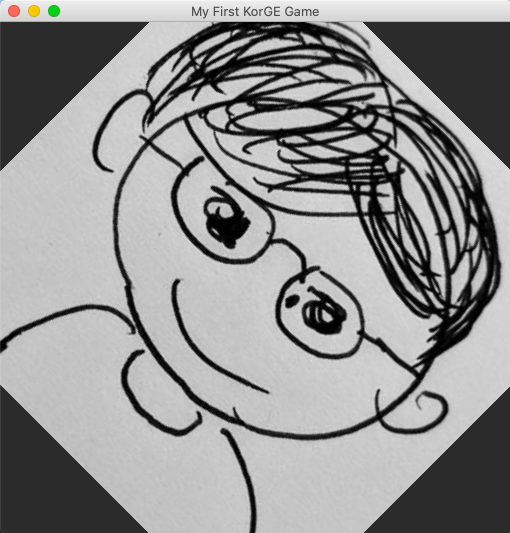#DAY 4
0
Software Development

## [Day04] 使用Image處理圖片

``````image(resourcesVfs["mylogo.png"].readBitmap())
``````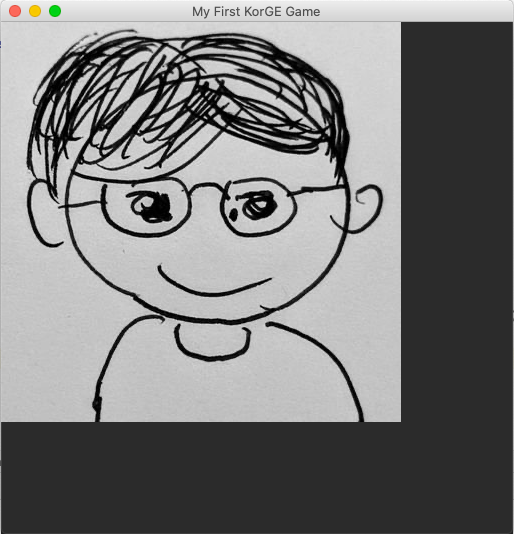Image.kt

``````inline fun Container.image(
texture: Bitmap, anchorX: Double = 0.0, anchorY: Double = 0.0, callback: @ViewDslMarker Image.() -> Unit = {}
): Image = Image(texture, anchorX, anchorY).addTo(this, callback)
``````

``````image(resourcesVfs["mylogo.png"].readBitmap()) {
width = ConfigModule.size.width.toDouble()
height = ConfigModule.size.height.toDouble()
}
``````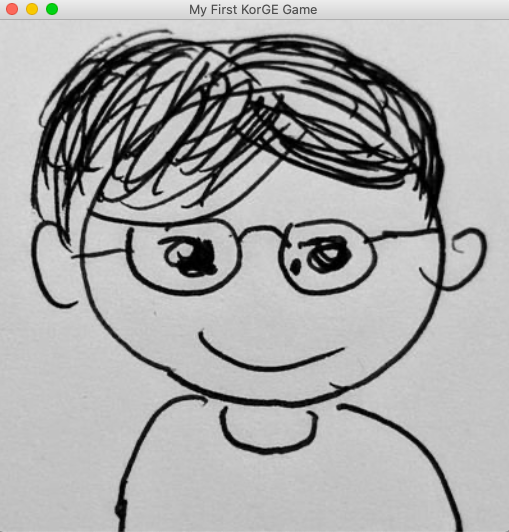``````val image = image(resourcesVfs["mylogo.png"].readBitmap()) {
width = ConfigModule.size.width.toDouble()
height = ConfigModule.size.height.toDouble()
position(100,100)
}
``````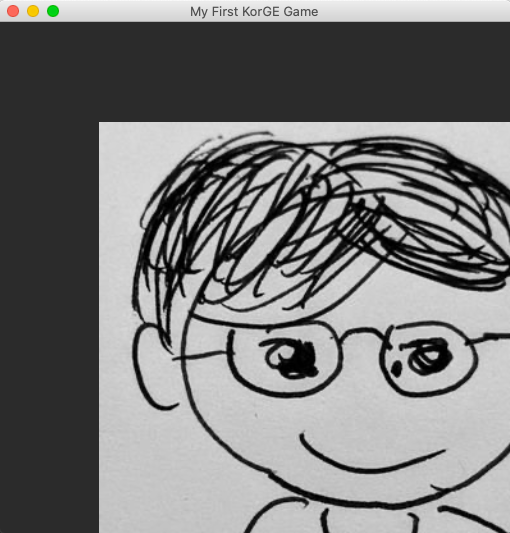``````image(resourcesVfs["mylogo.png"].readBitmap()) {
width = ConfigModule.size.width.toDouble()
height = ConfigModule.size.height.toDouble()
alpha = 0.5
}
``````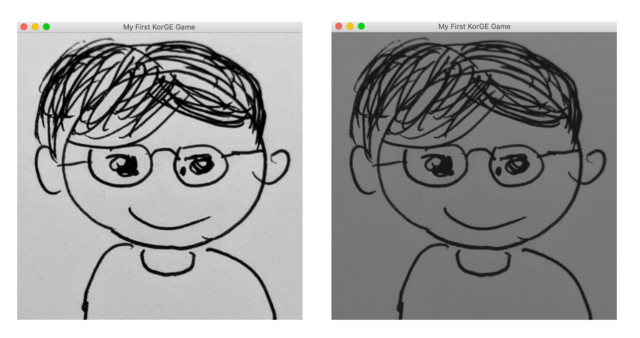``````image(resourcesVfs["mylogo.png"].readBitmap()) {
width = ConfigModule.size.width.toDouble()
height = ConfigModule.size.height.toDouble()
rotationDegrees = 45.0
}
``````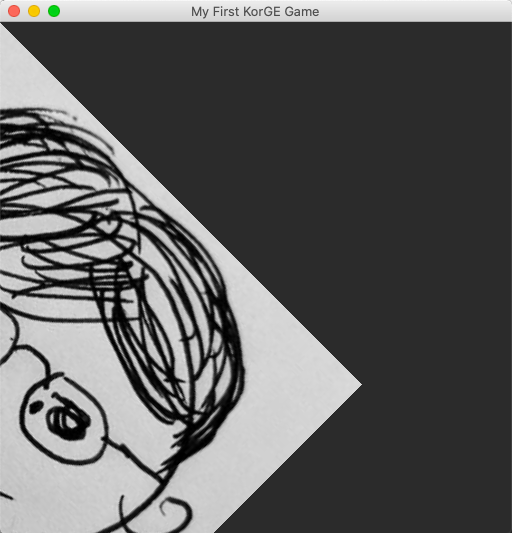``````image(resourcesVfs["mylogo.png"].readBitmap()) {
anchor(.5, .5)
width = ConfigModule.size.width.toDouble()
height = ConfigModule.size.height.toDouble()
rotationDegrees = 45.0
}
``````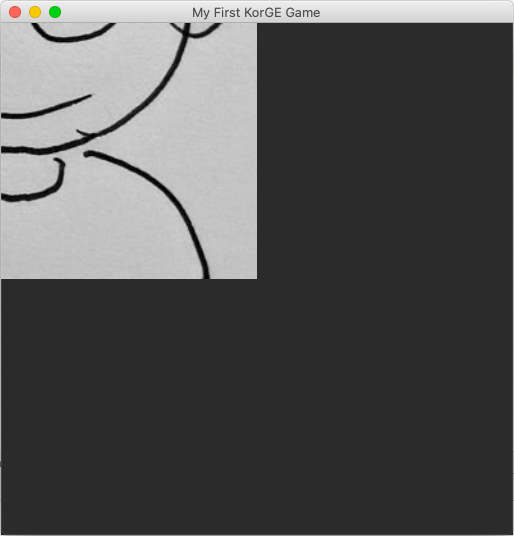``````image(resourcesVfs["mylogo.png"].readBitmap()) {
anchor(0.5, 0.5)
width = ConfigModule.size.width.toDouble()
height = ConfigModule.size.height.toDouble()
position(scaledWidth/2, scaledHeight/2)
}
``````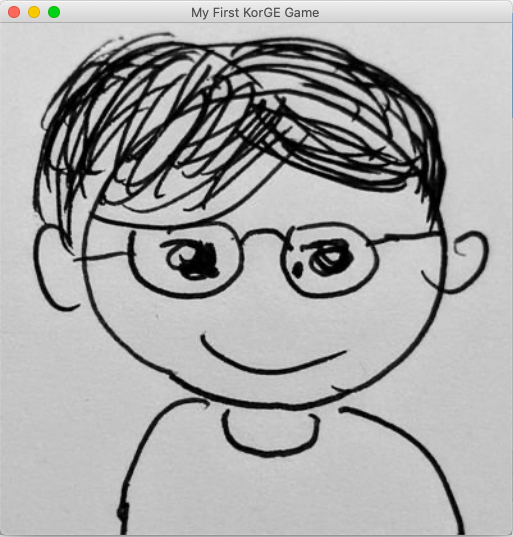``````image(resourcesVfs["mylogo.png"].readBitmap()) {
anchor(0.5, 0.5)
width = ConfigModule.size.width.toDouble()
height = ConfigModule.size.height.toDouble()
position(scaledWidth/2, scaledHeight/2)
rotationDegrees = 45.0
}
``````